Connect with us

Standard Costing Fundamental [Step-by-Step]Published

onStandard costing is a method of setting targets and reporting divergences from them. That is in short words. The process to do it isn’t really that short. In this post, I am going to reveal it, step-by-step, to make sure it is the easiest way to understand it. But before that, let me explain it in brief. Read on…

Financial reporting tells you how much profit or loss we have made in a period. Comparison with the budget will tell you whether you have surpassed budget or fallen short. For effective control of operations, you need much more information than that. You want to know how the company have performed against its expected costs for each type of item it produces.

It is necessary in the first place to establish suitable targets. This can be done theoretically, but it will be found that in practice such targets can be less accurate than required. Therefore targets are revised once an item goes into production, and may be revised at intervals as costs and methods change.

The implication here is that standard costing is most useful for environments where large numbers of a particular item are produced, typically manufacturing. In such an environment careful monitoring and control of variable costs can pay large dividends in terms of profit; and the variety of reasons for divergence from standard costs means that you need a formal system of analysis.

There is no reason in theory why standard costing could not be applied in an organization supplying standardized services in high volumes. However, typically variable costs are small compared with fixed costs so a formal system may cost more than it is worth.

Standards are set on the basis of realistic expectations, not best or worst cases, and they also rely on assumptions about the level of production usually a level at which there is little idle capacity. A typical standard will break down the components of a product into its material and processing costs in some detail.

Periodically the actual outcome will be analyzed and variances noted. I will examine an example in some depth, but the first point to note is that the causes of deviation from the expected profit are categorized in such a way as to make corrective action possible. A simple calculation of overall gain or loss against budget would not be very useful.

Flexed Budgets

Budgets are prepared at some particular point in time, before the start of the period to which they relate. The level of activity during the period how many items will be manufactured or how many customers will be served – cannot be known for certain and is estimated. This estimate will be based on a forecast or target and is set to fit in with other elements of the budget for the whole company.

After the end of the period, actual results will be compared in detail with the budget. Some of the differences will result from a difference in the actual activity level from the estimated level. Others will be due to differences in unit cost, for example in the cost of one kilo of materials or one hour of labor time.

A straightforward comparison between budget and results will tell you nothing about which differences (variances) are differences in activity level (volume variances) and which are due to differences in unit cost (cost variances). A variance analysis is carried out to break the variances down into more detail, distinguishing between production volume and cost variances.

Similarly, if the budget includes revenue, the difference between budget and actual revenue can be broken down into two parts. One set of variances result from more or less activity than planned, and the other set result from charging more or less than planned for goods or services.

How can all this be achieved? The key is to flex the budget.

The flexed budget is created by recalculating the original budget using the actual level of activity. This removes activity variances so they can be dealt with separately. So you end up with three statements: the original budget, the flexed budget, and the actual results. It helps to think about when these statements are prepared. The original budget (which will often be referred to simply as ‘the budget’) will have been prepared before the period in question.

How To Calculate Variances

It is easy to get confused by the many types of variance, and pointless trying to learn them by rote. Remember that there are many potentially useful variances; some are frequently used and it certainly helps to recognize these. But the result of a calculation is useful even if you don’t actually give the variance a recognized name.

Think: what am I trying to achieve with this variance? Am I looking at differences in quantity (of materials used, for example) or differences in price?

For variances in materials used in manufacture it is customary to use the expressions ‘price’ and ‘quantity’. For variances associated with labor such as hourly rate and hours worked – it is customary to use the expressions ‘rate’ and ‘efficiency’. But the arithmetic is identical. Let’s go with a simple example. Read on…

Variance Calculation Case Example

A very simple product is made up from cardboard shapes by hand. The worker who makes the boxes is paid by the hour. When the budget was drawn up for January, the target activity level was set at 3,600 boxes. At the end of January, the actual results are compared with the budget cost.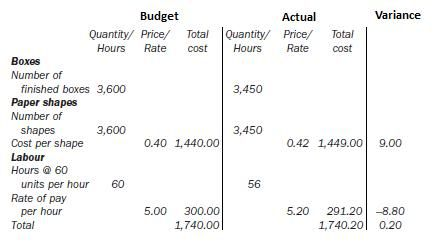At first sight, the result seems to be acceptable since the actual cost is almost identical to the budget cost. The materials have cost a little more and the labor has cost a little less, but the overall effect is very close to the estimate. However, further analysis shows that there are, in fact, adverse variances. The first step is to replace the original budget with a flexed budget. This is calculated as if an activity level of 3,450 units had been forecast in the first place. By convention, we label favorable variances ‘F’ and adverse variances ‘A’. Some prefer to use brackets for adverse variances and omit them for favorable ones.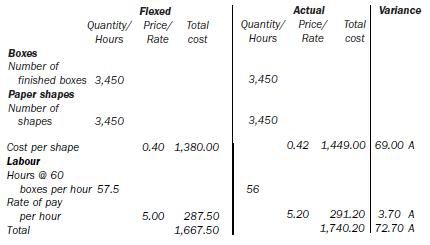Now you can see the situation more clearly. The paper shapes have cost more per unit than planned. The labor rate per hour has increased, but this effect has been partly offset by greater efficiency – the boxes have been made in slightly fewer hours than the standard. Overall, the boxes have cost \$72.70 more than expected. You can go further in our detective work to find out what happened.

How To Calculate Usage (efficiency) Variances

The number of cardboard shapes used (3,450) is exactly the number of shapes which should have been used, there was no waste. However, you would have expected the production level of 3,450 boxes to take 57.5 hours to complete (this is 3,450/60 units = 57.5). In fact production took 56 hours – a favorable outcome.

You can calculate the financial effect exactly; the standard labor rate is \$5.00 per hour so the variance is 1.5 hours at \$5 per hour which comes to a favorable variance of \$7.50. Note that people tend to use slightly different terms for labor and materials – the price is called a labor rate (not price) and the usage variance is called an efficiency variance.

How To Calculate Price (rate) Variances

The cardboard shapes were expected to cost 40 pence each but actually cost 42 pence each. The price variance is:

(0.42 – 0.40) × 3,450 = an adverse variance of \$69.00

What if the prices or rates had not been given per unit or per hour? This does not pose a problem – you still can work out the variances via total cost – but if you want to explain the price changes in terms of one unit or one hour, you will have to use the average. The labor rate was expected to be \$5.00 per hour but was actually \$5.20 per hour.

56 hours were worked so the rate variance is 56 × (5.20 – 5.00) = \$11.20 adverse.

You can check it; the sum of the individual variances should equal the overall adverse variance of 72.70.

Materials
Usage                0
Price           69.00
69.00
Labor
Efficiency    7.50
Rate         -11.20
-3.70
Total                   -72.70

Variances relating to those costs which are in some way variable with volume of activity can be analyzed in an easy way, though the terminology is slightly different. Variable expenditure variance and variable efficiency variance add up to total variable overhead variance. The calculation is identical to the usage and price variances above.

Fixed Costs

Fixed costs, of course, are not linked with volume of activity—by definition. However the amount expended may vary from budget and this needs to be taken into account somehow if actual profit is to be reconciled with budgeted profit. It is legitimate to simply state the under- or over-expenditure as a total variance.

Alternatively, this total variance can be broken down into two components, the volume and budget variances. The volume variance arises when the actual level of activity differs from the level assumed in the original budget. The budget variance arises when the actual cost differs from the cost justified by the actual level of activity.

How To Calculate Revenue Variances

Revenue can differ from budget for various reasons. For standard costing purposes, I can say that sales in volume terms may vary from budget or the prices charged may be different, or both. As you did for costs, these differences can be calculated and the terminology is that the sales (margin) volume variance and the sales price variance add up to the total sales variance.

Sales mix

Profit can also vary because the sales mix is different from planned products are sold in different proportions than planned. If the products have differing sales margins, this will make a difference to the profit.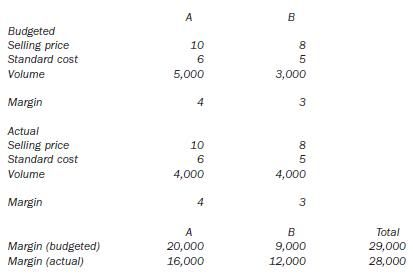The results show that the actual margin was \$1,000 less than budgeted, because of the sales mix – 1,000 units of product B have replaced 1,000 units of product A, but the sales margin on product B is \$1 lower.

How To Reconcile Profit

You need to reconcile actual and budgeted profit for several reasons. An underlying reason for using a standard costing system is to improve control; someone will be required to account for any shortfall in budgeted profit and will need to know the details of what happened. It is equally important to analyze what happened when we surpass the budget – again to control the system and, where appropriate, to recognize and reward success.

Every significant variation from the budget feeds back into the budget-setting process, letting you know how well our process works. If large variances recur, you may need to recognize that the environment has changed and you need a new forecast for the rest of the year; a quoted company may need to issue a profit warning or update, and any firm must be alive to the dangers outlined earlier of missing its cash forecast.

If you keep finding large fluctuations in variances, you may need to rethink your budget making process for the next year. Let’s go over an example on how to reconcile profit.

Profit Reconciliation Example

A company produces a product for which the standard costs are shown below. For January, the planned level of production is 5,000 units. Annual budgeted fixed overheads are \$600,000.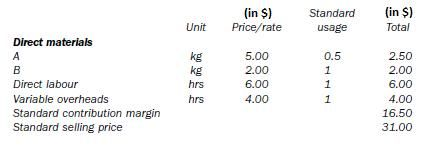Actual results for January were as follows: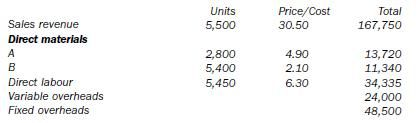The budget is flexed: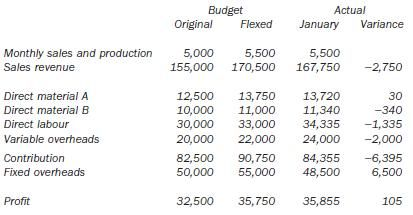And the variances can be calculated in more detail. Note that the totals should correspond – for example, for material A the two variances below are +280 and -250, totalling +30 to match the table above.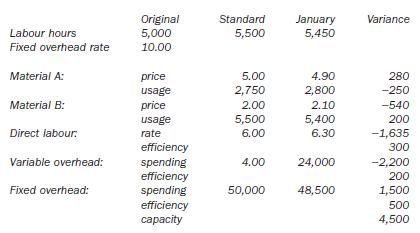Sales margin variances: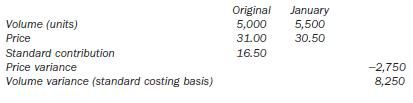Finally a report can be prepared if required, which reconciles budgeted profit with actual profit.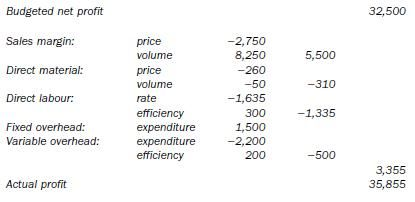Some people like to label variances ‘favorable’ and ‘adverse’ rather than just showing them as positive or negative. It really doesn’t matter, but pick a style and stick to it if your arithmetic is to work first time. If you remember that your standard costs plus your variances must equal the figures you were given to work with, you can hardly go far wrong.

Standard costing forms the basis for most systems used in manufacturing industry, and familiarity with these ideas is essential. In practice, there is no standard set of variances for all occasions. If you understand the fundamentals, then it is easy to calculate new variances that someone thinks may be useful. If your method is right, you will be rewarded even if you can’t remember the name for some of the variances you calculate.

Are you looking for easy accounting tutorial? Established since 2007, Accounting-Financial-Tax.com hosts more than 1300 articles (still growing), and has helped millions accounting student, teacher, junior accountants and small business owners, worldwide.